# Trigonometric function facts for kids

Kids Encyclopedia Facts
(Redirected from Trigonometric functions)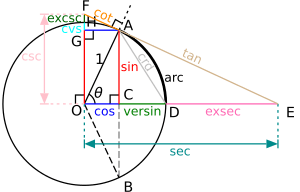All of the trigonometric functions of any angle can be constructed using a circle centered at O with radius of 1.

In mathematics, the trigonometric functions are a set of functions which relate angles to the sides of a right triangle. There are many trigonometric functions, the 3 most common being sine, cosine, tangent, followed by cotangent, secant and cosecant. The last three are called reciprocal trigonometric functions, because they act as the reciprocals of other functions. Secant and cosecant are rarely used.

 Function Abbreviation Relation Sine sin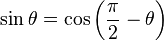$\sin \theta = \cos \left(\frac{\pi}{2} - \theta \right) \,$ Cosine cos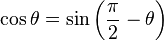$\cos \theta = \sin \left(\frac{\pi}{2} - \theta \right)\,$ Tangent tan (or tg)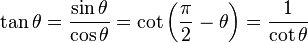$\tan \theta = \frac{\sin \theta}{\cos \theta} = \cot \left(\frac{\pi}{2} - \theta \right) = \frac{1}{\cot \theta} \,$ Cotangent cot (or ctg)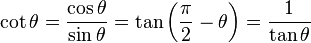$\cot \theta = \frac{\cos \theta}{\sin \theta} = \tan \left(\frac{\pi}{2} - \theta \right) = \frac{1}{\tan \theta} \,$ Secant sec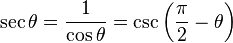$\sec \theta = \frac{1}{\cos \theta} = \csc \left(\frac{\pi}{2} - \theta \right) \,$ Cosecant csc (or cosec)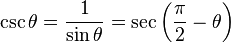$\csc \theta =\frac{1}{\sin \theta} = \sec \left(\frac{\pi}{2} - \theta \right) \,$

## Definition

The trigonometric functions sometimes are also called circular functions. They are functions of an angle; they are important when studying triangles, among many other applications. Trigonometric functions are commonly defined as ratios of two sides of a right triangle containing the angle, and can equivalently be defined as the lengths of various line segments from a unit circle (a circle with radius of one).

### Right triangle definitions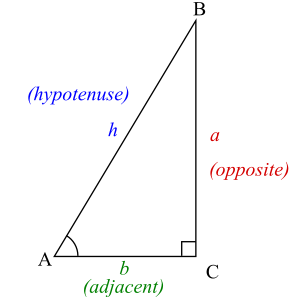A right triangle always includes a 90° (π/2 radians) angle, here labeled C. Angles A and B may vary. Trigonometric functions specify the relationships between side lengths and interior angles of a right triangle.

In order to define the trigonometric functions for the angle A, start with a right triangle that contains the angle A:

We use the following names for the sides of the triangle:

• The hypotenuse is the side opposite the right angle, also the longest side of a right-angled triangle, in this case h.
• The opposite side is the side opposite to the angle we are interested in, in this case a.
• The adjacent side is the side that is in contact with the right angle the angle we are interested in, hence its name. In this case, the adjacent side is b.

All triangles are taken to exist in Euclidean geometry, so that the inside angles of each triangle sum to π radians (or 180°); therefore, for a right triangle, the two non-right angles are between zero and π/2 radians. Notice that strictly speaking, the following definitions only define the trigonometric functions for angles in this range. We extend them to the full set of real arguments by using the unit circle, or by requiring certain symmetries and that they be periodic functions.

1) The sine of an angle is the ratio of the length of the opposite side to the length of the hypotenuse. In our case$\sin A = \frac {\textrm{opposite}} {\textrm{hypotenuse}} = \frac {a} {h}.$

Note that since all those triangles are similar, this ratio does not depend on the particular right triangle that is chosen, as long as it contains the angle A.

The set of zeroes of sine (that is, the values of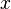$x$ for which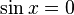$\sin x =0$) is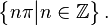$\left\{n\pi\big| n\isin\mathbb{Z}\right\}.$

2) The cosine of an angle is the ratio of the length of the adjacent side to the length of the hypotenuse. In our case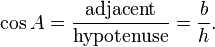$\cos A = \frac {\textrm{adjacent}} {\textrm{hypotenuse}} = \frac {b} {h}.$

The set of zeroes of cosine is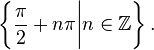$\left\{\frac{\pi}{2}+n\pi\bigg| n\isin\mathbb{Z}\right\}.$

3) The tangent of an angle is the ratio of the length of the opposite side to the length of the adjacent side. In our case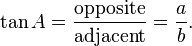$\tan A = \frac {\textrm{opposite}} {\textrm{adjacent}} = \frac {a} {b}.$

The set of zeroes of tangent is$\left\{n\pi\big| n\isin\mathbb{Z}\right\}.$

This is the same set as that of the sine function, since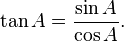$\tan A = \frac {\sin A}{\cos A}.$

The remaining three functions are best defined using the above three functions.

4) The cosecant csc(A) is the multiplicative inverse of sin(A); it is the ratio of the length of the hypotenuse to the length of the opposite side: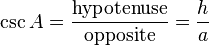$\csc A = \frac {\textrm{hypotenuse}} {\textrm{opposite}} = \frac {h} {a}$.

5) The secant sec(A) is the multiplicative inverse of cos(A); it is the ratio of the length of the hypotenuse to the length of the adjacent side: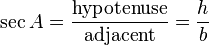$\sec A = \frac {\textrm{hypotenuse}} {\textrm{adjacent}} = \frac {h} {b}$.

6) The cotangent cot(A) is the multiplicative inverse of tan(A); it is the ratio of the length of the adjacent side to the length of the opposite side: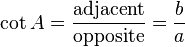$\cot A = \frac {\textrm{adjacent}} {\textrm{opposite}} = \frac {b} {a}$.

### Definitions by power series

One can also define the trigonometric functions by using power series: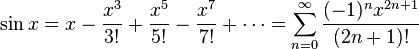$\sin x = x - \frac{x^3}{3!} + \frac{x^5}{5!} - \frac{x^7}{7!} + \cdots = \sum_{n=0}^\infty \frac{(-1)^nx^{2n+1}}{(2n+1)!}$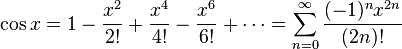$\cos x = 1 - \frac{x^2}{2!} + \frac{x^4}{4!} - \frac{x^6}{6!} + \cdots = \sum_{n=0}^\infty \frac{(-1)^nx^{2n}}{(2n)!}$

and define tangent, cotangent, secant and cosecant using identities, see below.

## Identities

Some important identities: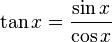$\tan x = \frac{\sin x}{\cos x}$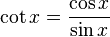$\cot x = \frac{\cos x}{\sin x}$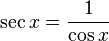$\sec x = \frac{1}{\cos x}$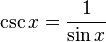$\csc x = \frac{1}{\sin x}$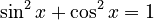$\sin^2 x + \cos^2 x = 1$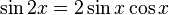$\sin 2x = 2 \sin x \cos x$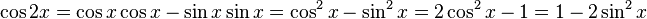$\cos 2x = \cos x \cos x - \sin x \sin x = \cos^2 x - \sin^2 x = 2 \cos^2 x - 1 = 1 - 2 \sin^2 x$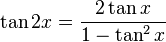$\tan 2x = \frac{2 \tan x}{1 - \tan^2 x}$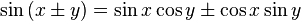$\sin \left(x \pm y \right)=\sin x \cos y \pm \cos x \sin y$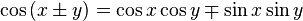$\cos \left(x \pm y \right)=\cos x \cos y \mp \sin x \sin y$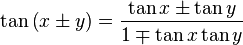$\tan \left ( x \pm y \right ) = \frac{\tan x \pm \tan y}{1 \mp \tan x \tan y}$

## Hyperbolic functions

The hyperbolic functions are like the trigonometric functions, in that they have very similar properties. Each of six trigonometric functions has a corresponding hyperbolic form. They are defined in terms of the exponential function, which is based on the constant e.

• Hyperbolic sine: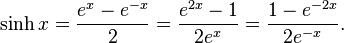$\sinh x = \frac {e^x - e^{-x}} {2} = \frac {e^{2x} - 1} {2e^x} = \frac {1 - e^{-2x}} {2e^{-x}}.$
• Hyperbolic cosine: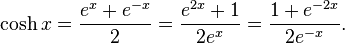$\cosh x = \frac {e^x + e^{-x}} {2} = \frac {e^{2x} + 1} {2e^x} = \frac {1 + e^{-2x}} {2e^{-x}}.$
• Hyperbolic tangent: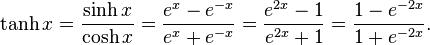$\tanh x = \frac{\sinh x}{\cosh x} = \frac {e^x - e^{-x}} {e^x + e^{-x}} = \frac{e^{2x} - 1} {e^{2x} + 1} = \frac{1 - e^{-2x}} {1 + e^{-2x}}.$
• Hyperbolic cotangent: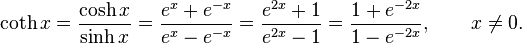$\coth x = \frac{\cosh x}{\sinh x} = \frac {e^x + e^{-x}} {e^x - e^{-x}} = \frac{e^{2x} + 1} {e^{2x} - 1} = \frac{1 + e^{-2x}} {1 - e^{-2x}}, \qquad x \neq 0.$
• Hyperbolic secant: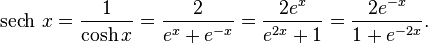$\operatorname{sech}\,x = \frac{1}{\cosh x} = \frac {2} {e^x + e^{-x}} = \frac{2e^x} {e^{2x} + 1} = \frac{2e^{-x}} {1 + e^{-2x}}.$
• Hyperbolic cosecant: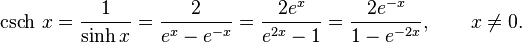$\operatorname{csch}\,x = \frac{1}{\sinh x} = \frac {2} {e^x - e^{-x}} = \frac{2e^x} {e^{2x} - 1} = \frac{2e^{-x}} {1 - e^{-2x}}, \qquad x \neq 0.$

## Related pages

• Joseph, George G., The Crest of the Peacock: Non-European Roots of Mathematics, 2nd ed. Penguin Books, London. (2000). .
• Weisstein, Eric W., "Tangent" from MathWorld, accessed 21 January 2006.

## Images for kidsTrigonometric function Facts for Kids. Kiddle Encyclopedia.